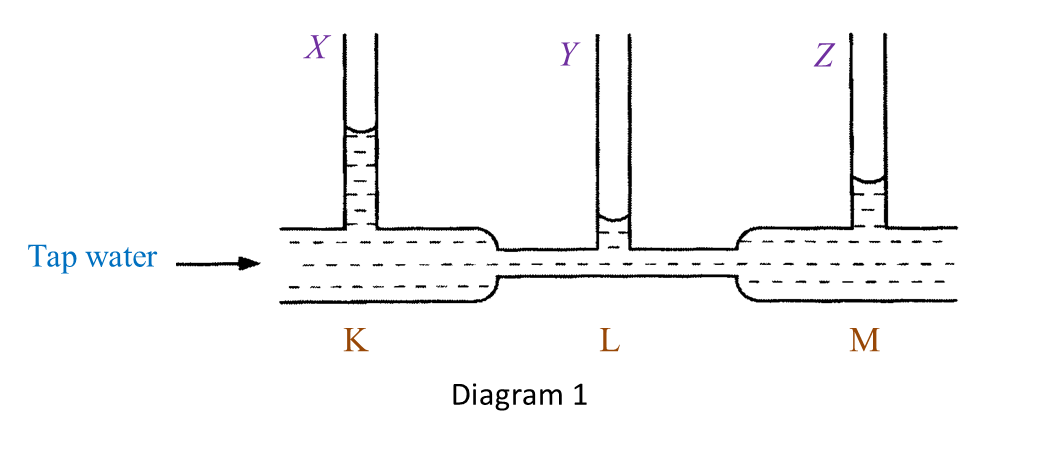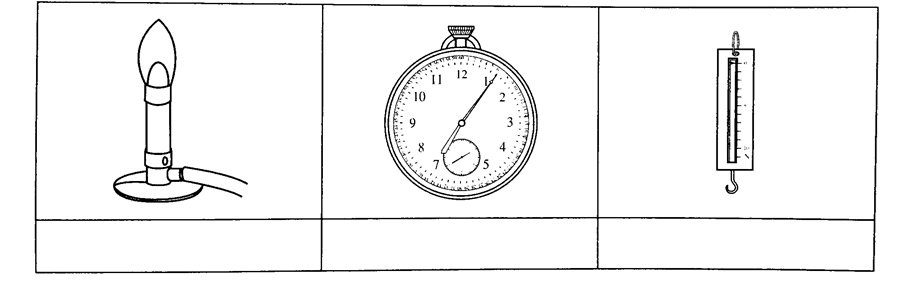# 5.7.4 The Motion of Vehicles in the Air (Structured Questions)

Question 1:
Diagram 1 shows an experiment to study Bernoulli’s Principle.(a)(i) State one observation of the water level in Diagram 1. [1 mark]

(ii) State one inference that can be made based on the observation in (a)(i). [1 mark]

(b) State the responding variable in this experiment. [1 mark]

(c) Based on this experiment, state the operational definition for Bernoulli’s Principle. [1 mark]

(d) Mark (\/) in the boxes provided to show the apparatus where the Bernoulli’s Principle is applied. [1 mark](a)(i)
– The water level in the vertical tube Y is the lowest.
– The water level in the vertical tube X is the highest.
(any one)

(a)(ii)
– The water at the base of the vertical tube Y exerts the lowest pressure.
– The water at the base of the vertical tube X exerts the highest pressure.
(any one)

(b)
The pressure exerted by the moving water.

(c)
Bernoulli’s Principle states that pressure in a liquid decreases when the speed of the liquid increases.

(d)